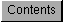This chapter describes the structure and calling sequences required to
access the Motorola Fast Floating Point (FFP), the IEEE single-precision
math libraries and the IEEE double-precision math libraries via the
Amiga-supplied interfaces.

In its present state, the FFP library consists of three separate entities:
the basic math library, the transcendental math library, and C and
assembly-language interfaces to the basic math library plus FFP conversion
functions.  The IEEE single-precision, introduced in Release 2, and the
double-precision libraries each presently consists of two entities: the
basic math library and the transcendental math library.

Open Each Library Separately.
-----------------------------
Each Task using an IEEE math library must open the library itself.
Library base pointers to these libraries may not be shared.
Libraries can be context sensitive and may use the Task structure to
keep track of the current context.  Sharing of library bases by Tasks
may seem to work in some systems.  This is true for any of the IEEE
math libraries.

Depending on the compiler used, it is not always necessary to explicitly
call the library functions for basic floating point operations as adding,
subtracting, dividing, etc.  Consult the manual supplied with the compiler
for information regarding the compiler options for floating point
functions.

Math Libraries and Functions
FFP Floating Point Data Format
FFP Basic Mathematics Library
FFP Transcendental Mathematics Library
FFP Mathematics Conversion Library
IEEE Single-Precision Data Format
IEEE Single-Precision Basic Math Library
IEEE Single-Precision Transcendental Math Library
IEEE Double-Precision Data Format
IEEE Double-Precision Basic Math Library
IEEE Double-Precision Transcendental Math Library
Function Reference
Compile and Link Commands for SAS C 5.10

[Back to Amiga Developer Docs]